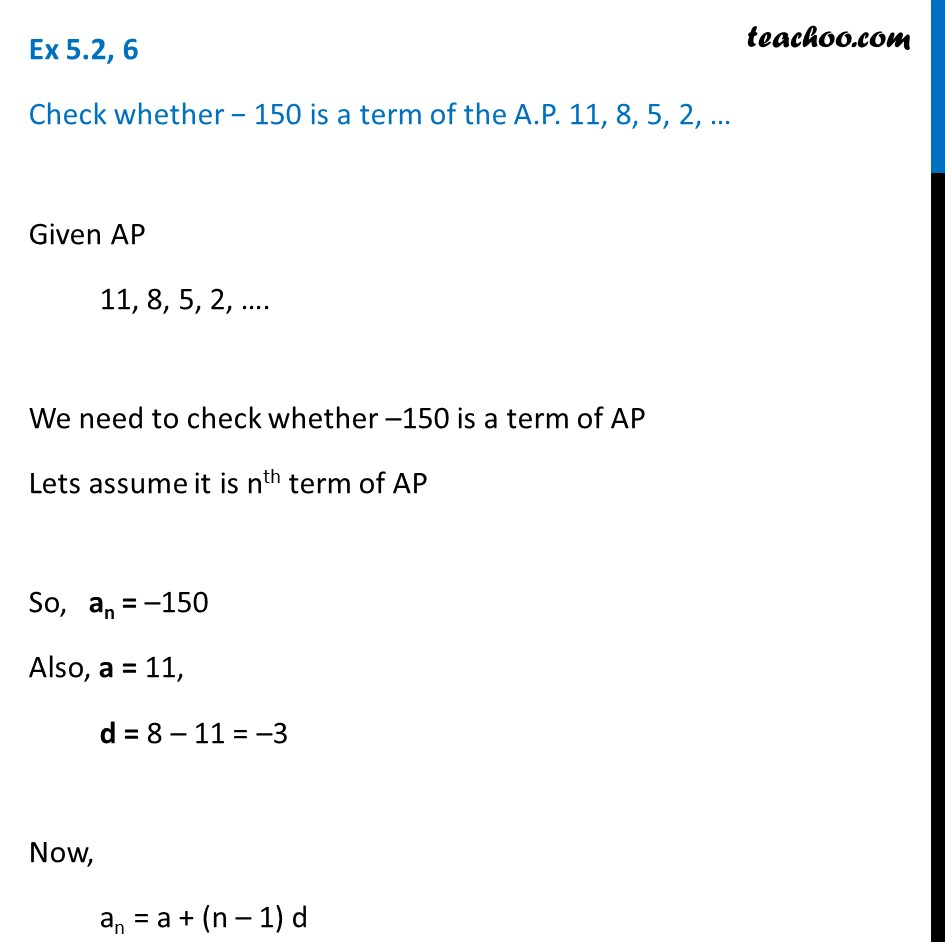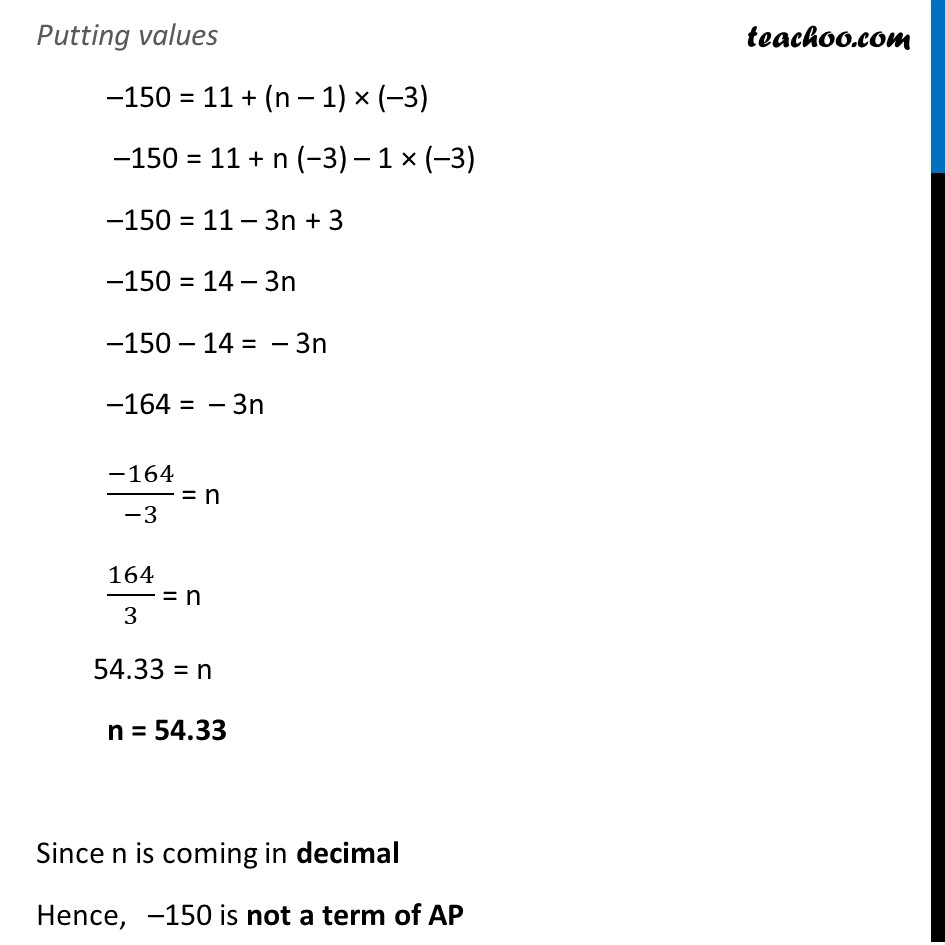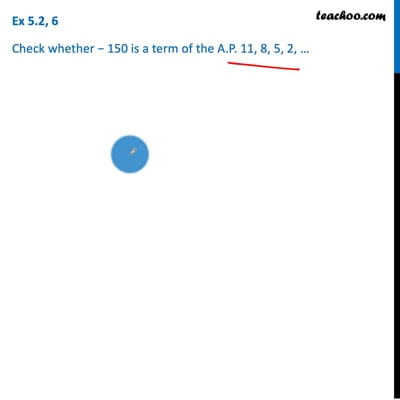Ex 5.2

Chapter 5 Class 10 Arithmetic Progressions
Serial order wiseThis video is only available for Teachoo black users

Introducing your new favourite teacher - Teachoo Black, at only ₹83 per month

### Transcript

Ex 5.2, 6 Check whether − 150 is a term of the A.P. 11, 8, 5, 2, … Given AP 11, 8, 5, 2, …. We need to check whether –150 is a term of AP Lets assume it is nth term of AP So, an = –150 Also, a = 11, d = 8 – 11 = –3 Now, an = a + (n – 1) d Putting values –150 = 11 + (n – 1) × (–3) –150 = 11 + n (−3) – 1 × (–3) –150 = 11 – 3n + 3 –150 = 14 – 3n –150 – 14 = – 3n –164 = – 3n (−164)/(−3) = n 164/3 = n 54.33 = n n = 54.33 Since n is coming in decimal Hence, –150 is not a term of AP